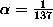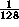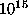The Net Advance of Physics: The Nature of Dark Matter, by Kim Griest -- Section 6B.

Next: Relic Abundance Up: Abstract Previous: Search for Wimp

## Motivation for Supersymmetry

First, why should astrophysicists take seriously supersymmetry, a

theory which requires more than doubling the number of known

elementary particles, none of which has yet been detected? When

Dirac attempted to make special relativity consistent with quantum

mechanics he discovered the Dirac equation. He also discovered a

disconcerting fact: there was a new symmetry, CPT symmetry,

implied by his equation, and this symmetry required that for every

known particle there had to exist a charge conjugate, or

anti-particle. He resisted the idea of doubling the number of known

particles, and initially hypothesized that the CPT partner of the

electron was the proton. This idea was soon shown to be impossible,

but fortunately the anti-electron was soon discovered, vindicating

Dirac's theory.

The situation may be similar with regard to supersymmetry. Many

attempts have been made to make general relativity consistent with

quantum field theory, especially within the framework of a theory

which combines gravity with the strong and electroweak

interactions. It is interesting that in all the most successful attempts

a new symmetry is required. The powerful Coleman-Mandula

theorem states that within the framework of Lie algebras, there is

no way to unify gravity with the gauge symmetries which describe

the strong and electroweak interactions. So the ``super"-symmetry

which successfully combines these interactions had to move beyond

like Lie algebras except they use anti-commutation relations

instead of commutation relations. Thus they relate particles with

spin to particles without spin. Examples of theories that attempt to

combine gravity with the other forces include super-strings and

super-gravity, where in both cases ``super" refers to the

supersymmetry. Thus, if such a symmetry exists in nature, every

particle with spin (fermion) must have a related super-symmetric

partner without spin (boson), and vice versa.

As it now stands, standard quantum field theory seems to be

incompatible with general relativity. Since the world is unlikely to

be incompatible with itself, it seems either quantum field theory or

general relativity must be modified, and of course a new theory

which combines gravity with the strong and electroweak

interactions would be the most elegant. Thus, one sees why so many

particle physicists have become enamored with supersymmetry, and

why many thousands of papers have been written on the subject.

As in Dirac's case, this doubling of the number of particles was

disconcerting, and it was initially hoped that perhaps the neutrino

could be the supersymmetric partner of the photon. Now it is known

that this is impossible, but in contrast to Dirac's case, no discovery

of supersymmetric partners has quickly followed. In fact, it is now

known that supersymmetry must be a ``broken" symmetry, since

perfect supersymmetry requires that the masses of the

super-partners be the same as their counterparts. This is easily

arranged, but leaves the masses of all the superpartners

undetermined. In fact, the masses could be so large that all the

superpartners are completely undetectable in current or future

accelerators and are therefore mostly irrelevant to current physics

or dark matter detection. There are however some very suggestive

reasons why the superpartners may have masses in the 100 GeV to

several TeV range.

First, there is coupling constant unification. The strength of the

strong, weak, and electromagnetic interactions is set by the value of

their coupling constants, and these ``constants" change as the

energy of the interactions increase. For example, the

electromagnetic coupling constant, has a value nearwhen electrons are collided at the LEP machine at CERN. Several

decades ago it was noticed that the three coupling constants would

meet together at a universal value when the energy of interactions

reached aboutGeV. This would allow a ``Grand Unification" of

the strong, weak, and electromagnetic interactions, and much

model building was done. In the past few years, the values of the

three coupling constants have been measured much more

accurately, and it is now clear that, in fact, they cannot unify at any

scale unless many new particles are added to the theory.

Suggestively, if the supersymmetric partners exist, and have

reasonably low masses, they give just the right contribution to force

the coupling constants to unify.

Next, there is the gauge hierarchy problem. The standard model of

particle physics is enormously successful. It accurately predicts the

results of hundreds of measurements. In the standard model,

fermions such as electrons are intrinsically massless, but develop

a mass through interactions with the Higgs field that is hypothesized

to fill the Universe. The mass of a fermion then is just proportional

to the strength of its coupling to the Higgs field. The Higgs is thus

an essential feature of the standard model. The Higgs also develops

a mass through a ``bare" mass term and interactions with other

particles, but due to its scalar nature, the mass it acquires through

interactions are as large as the largest mass scale in the theory.

Thus, in any unified model, the Higgs mass tends to be enormous.

Such a large Higgs mass cannot be, however, since it would ruin the

successful perturbation expansion used in all standard model

calculations. Thus in order to get the required low Higgs mass, the

bare mass must be fine-tuned to dozens of significant places in

order to precisely cancel the very large interaction terms. At each

order of the perturbation expansion (loop-expansion), the

procedure must be repeated.

However, if supersymmetric partners are included, this fine-tuning

is not needed. The contribution of each supersymmetric partner

cancels off the contribution of each ordinary particle. This works

only if the supersymmetric partners have masses below the TeV

range. Thus, stabilization of the gauge hierarchy is accomplished

automatically, as long as supersymmetric particles exist and have

masses in the range 100 -1000 GeV. The enormous effort going

into searches for supersymmetric particles at CERN, Fermilab, etc.

is largely motivated by this argument.

Even though no supersymmetric particles have been discovered,

they have all been given names. They are named after their

partners. Bosonic ordinary particles have fermonic superpartners

with the same name except with the suffix ``ino" added, while

fermonic ordinary particles have bosonic (scalar) superpartner

names with the prefix ``s" added. So for example, the photino,

Higgsino, Z-ino, and gluino are the partners of the photon, Higgs,

Z-boson, and gluon respectively. And the squark, sneutrino, and

selectron are the scalar superpartners of the quark, neutrino, and

electron respectively. There are several superpartners which have

the same quantum numbers and so can mix together in linear

combinations. Since these do not necessarily correspond to any one

ordinary particle, they are given different names. For example, the

photino, Higgsino, and Z-ino can mix into arbitrary combinations

called the neutralinos, and the charged W-ino and charged

Higgsino combine into particles called charginos.

Finally, an interesting feature of most supersymmetric models is the

existence of a multiplicatively conserved quantum number called

R-parity, in which each superpartner is assigned R=-1, and each

ordinary particle is assigned R=+1. This quantum number implies

that supersymmetric particles must be created or destroyed in pairs,

and that the lightest supersymmetric particle (LSP) is absolutely

stable; just as the electron is stable since electric charge is conserved

and there is no lighter charged particle into which it could decay.

This fact is what makes supersymmetric particles dark matter

candidates. If supersymmetry exists and R-parity is conserved,

then some LSP's must exist from the Early Universe. The only

question is how many.Next: Relic Abundance Up: Abstract Previous: Search for Wimp